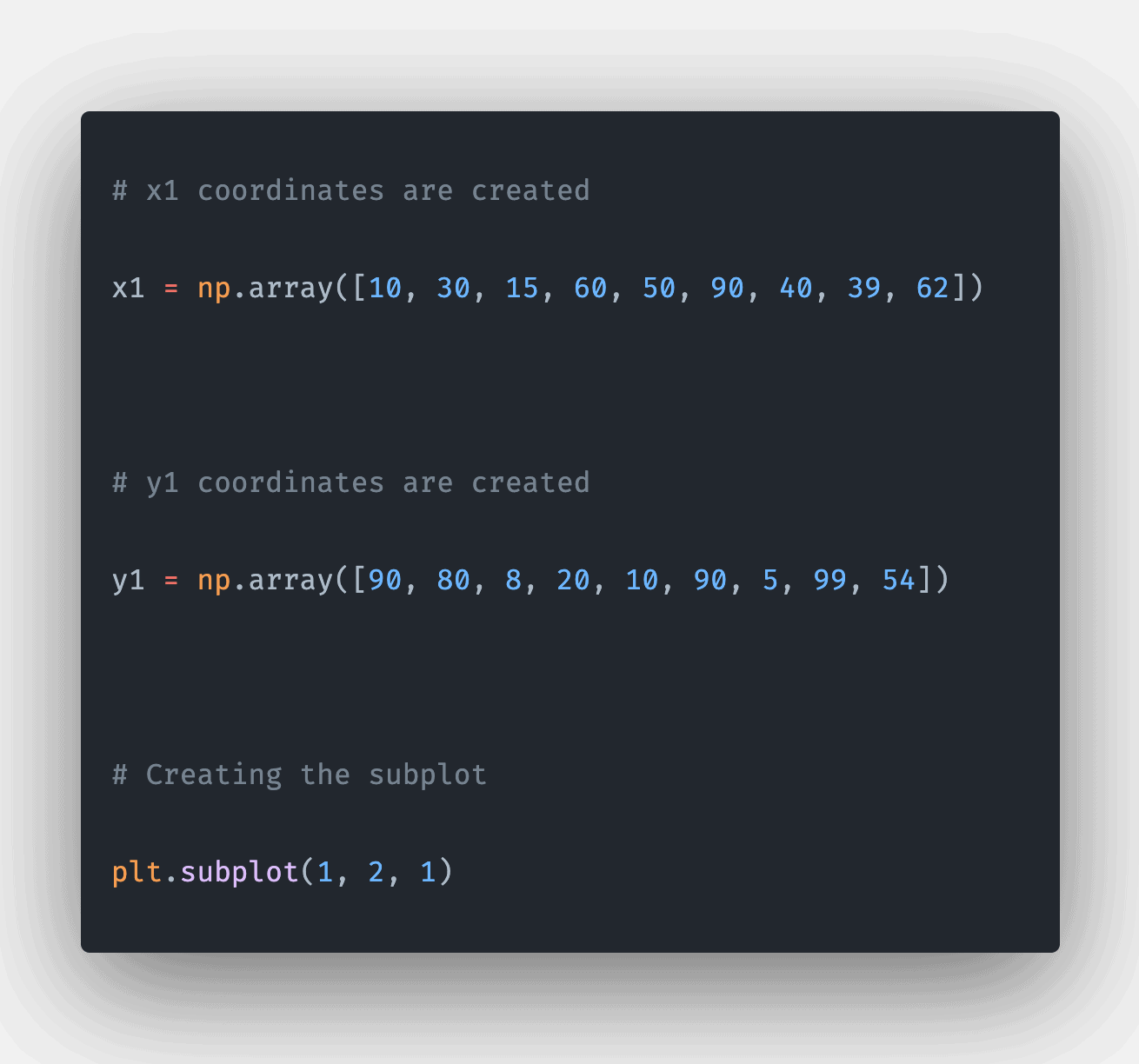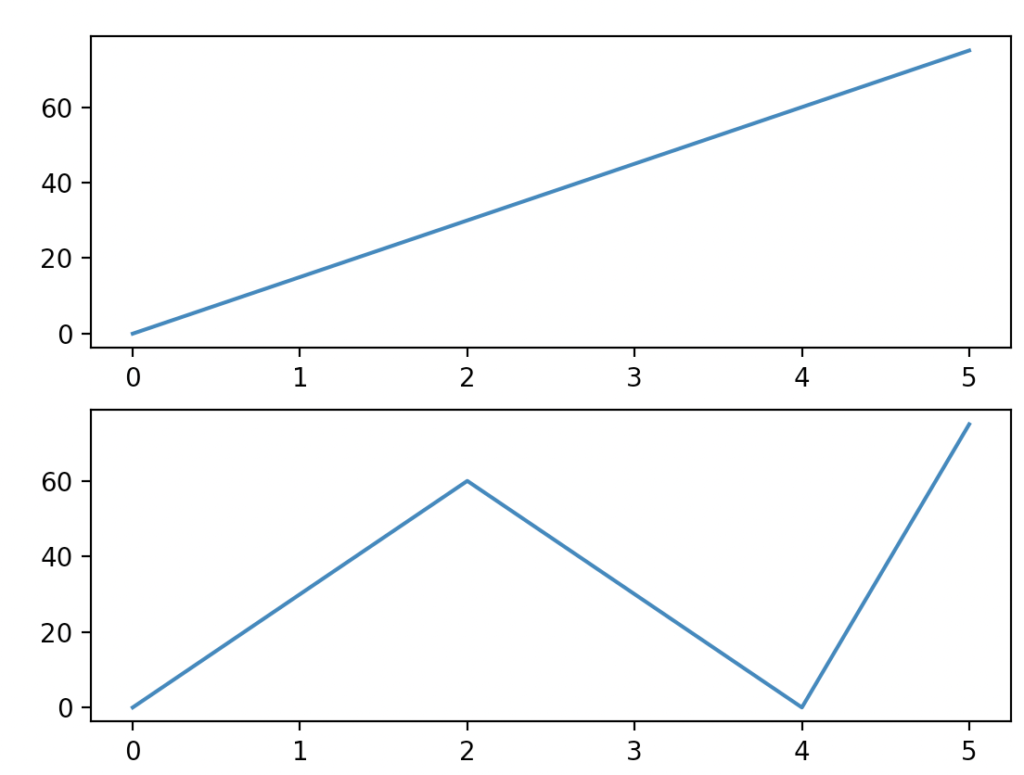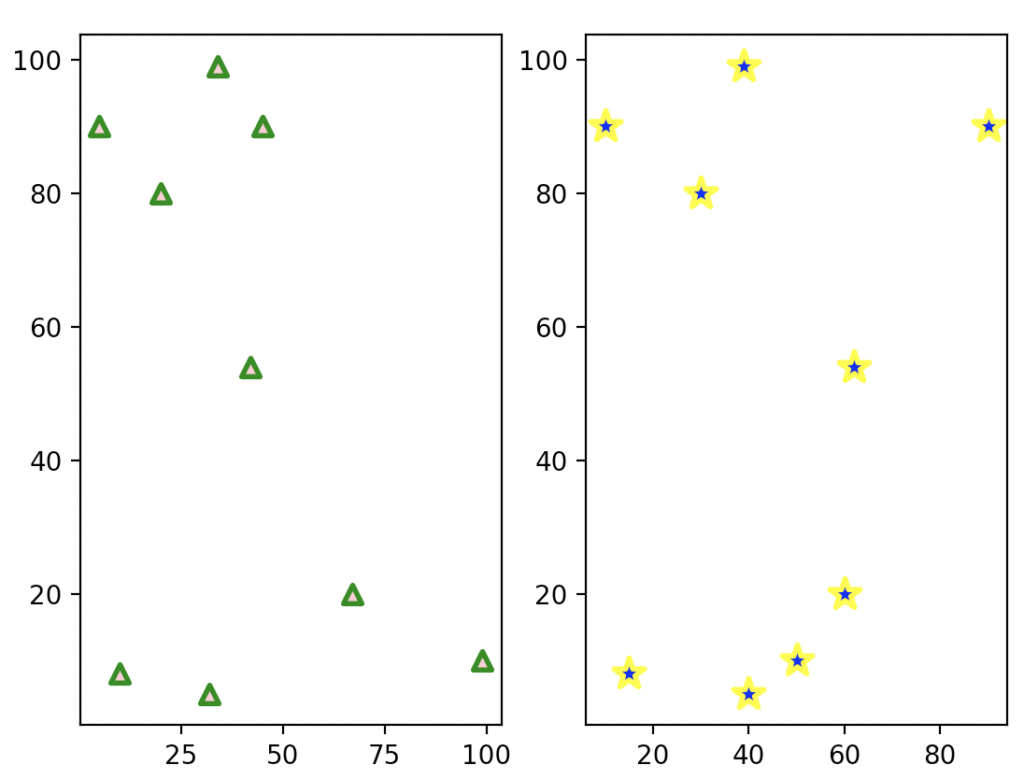# Matplotlib subplots: The Complete Guide

0
5The matplotlib.pyplot.subplots() function is a utility wrapper that makes it convenient to create standard layouts of subplots, including the enclosing figure object, in a single call.

## Matplotlib subplots

Matplotlib.pyplot.subplots() is a built-in library function that creates a subplot for the given points and displays the graph as the output. This function plots several graphs inside a single graph. The subplots() function creates a subplot inside a single plot.

### Syntax

``````matplotlib.pyplot.subplots(nrows=1, ncols=1,sharex=False, sharey=False, squeeze=True,
subplot_kw=None, gridspec_kw=None, **fig_kw) ``````

### Parameters

The matplotlib.pyplot.subplots() function arguments are optional:

1. nrows, ncols: The number of rows and columns are passed inside this argument. By default, it is kept as 1.
2. sharex, sharey: This takes a Boolean value. If True, it shares the corresponding x and y-axis. This also takes values like all, none, row, col. By default, it is assigned as False.
3. squeeze: This argument takes the Boolean value as the value. If True, the extra dimensions are removed if those dimensions are not used. If False, then this function always returns a 2D array. By default, the value is passed as True.
4. subplot_kw: This argument takes a dictionary as a value. The dict is passed with the keywords for adding a subplot into the plot.
5. gridspec_kw: This argument takes a dictionary as a value. The dict is passed with the keywords for creating the grid where the subplots are placed.

### Return value

The matplotlib.pyplot.subplots() function returns a figure, and it returns an array. This array consists of axes.

### Program for creating subplots using matplotlib.pyplot.subplot

``````# Importing matplotlib.pyplot as plt.
import matplotlib.pyplot as plt

# Importing numpy as np
import numpy as np

# create a numpy array for storing the x coordinates
x = np.array([0, 1, 2, 3, 4, 5])

# create a numpy array for storing the y coordinates
y = np.array([0, 15, 30, 45, 60, 75])

# create a numpy array for storing the x1 coordinates
x1 = np.array([0, 1, 2, 3, 4, 5])

# create a numpy array for storing the y1 coordinates
y1 = np.array([0, 30, 60, 30, 0, 75])

# subplot is created
plt.subplot(2, 1, 1)

# pass the x coordinates and y coordinates into the plot() function
plt.plot(x, y)
plt.subplot(2, 1, 2)

# pass the x1 coordinates and y1 coordinates into the plot() function
plt.plot(x1, y1)

# displaying the created graph using the show method
plt.show()``````

#### OutputIn this program, we have matplotlib.pyplot for plotting the subplot. The matplotlib library consists of all the functions for plotting different types of graphs and charts.

We imported numpy for creating x coordinates and y coordinates. Then, we created other points for plotting the second plot.

We have created a subplot with 2 rows. The axes for the 1st plot are kept as 1st row and 1st column. Then, we passed the x and y coordinates into the plot function. This function creates a straight line by combining x and y coordinates.

Then we changed the subplot axes to the second row and first column. Then we have displayed the plot using the show function. In this example, we have created two plots in a single plot.

### Program for creating two scatter plots in a subplot using matplotlib.pyplot.subplot

``````# Importing matplotlib.pyplot as plt.
import matplotlib.pyplot as plt

# Importing numpy as np
import numpy as np

# x coordinates are created
x = np.array([5, 20, 10, 67, 99, 45, 32, 34, 42])

# y coordinates are created
y = np.array([90, 80, 8, 20, 10, 90, 5, 99, 54])

# x1 coordinates are created
x1 = np.array([10, 30, 15, 60, 50, 90, 40, 39, 62])

# y1 coordinates are created
y1 = np.array([90, 80, 8, 20, 10, 90, 5, 99, 54])

# Creating the subplot
plt.subplot(1, 2, 1)

# scatter plot is created
plt.scatter(x, y, c="pink",
linewidths=2,
marker="^",
edgecolor="green",
s=50)
plt.subplot(1, 2, 2)
plt.scatter(x1, y1, c="blue",
linewidths=2,
marker="*",
edgecolor="yellow",
s=150)

# displaying the created graph using the show method
plt.show()``````

#### OutputIn this program, we imported matplotlib.pyplot to plot the scatter plot and create the subplot.

The matplotlib library consists of all the functions for plotting different types of graphs and charts. Then, we imported numpy for creating x coordinates and y coordinates.

Then we have created another set of points called x1 and y1. We created a subplot with axes as 1st row and 1st column. Then we passed x and y coordinates into the scatter function with the color pink, the edgecolor green, and the marker as a triangle.

This creates a scatter plot in the 1st row and 1st column. Then the subplot is changed to the axes as 1st row and 2nd column. Then we have passed the points x1 and y1 into the scatter function with the color as blue and the edge color as yellow, and the marker as a star. This creates a subplot in the 1st row and 2nd column.

Then we used the show function to display the generated scatter plot. In this example, two scatterplots are plotted in a single plot.

That’s it for this tutorial.

## Related posts

Matplotlib legend

Matplotlib histogram

This site uses Akismet to reduce spam. Learn how your comment data is processed.